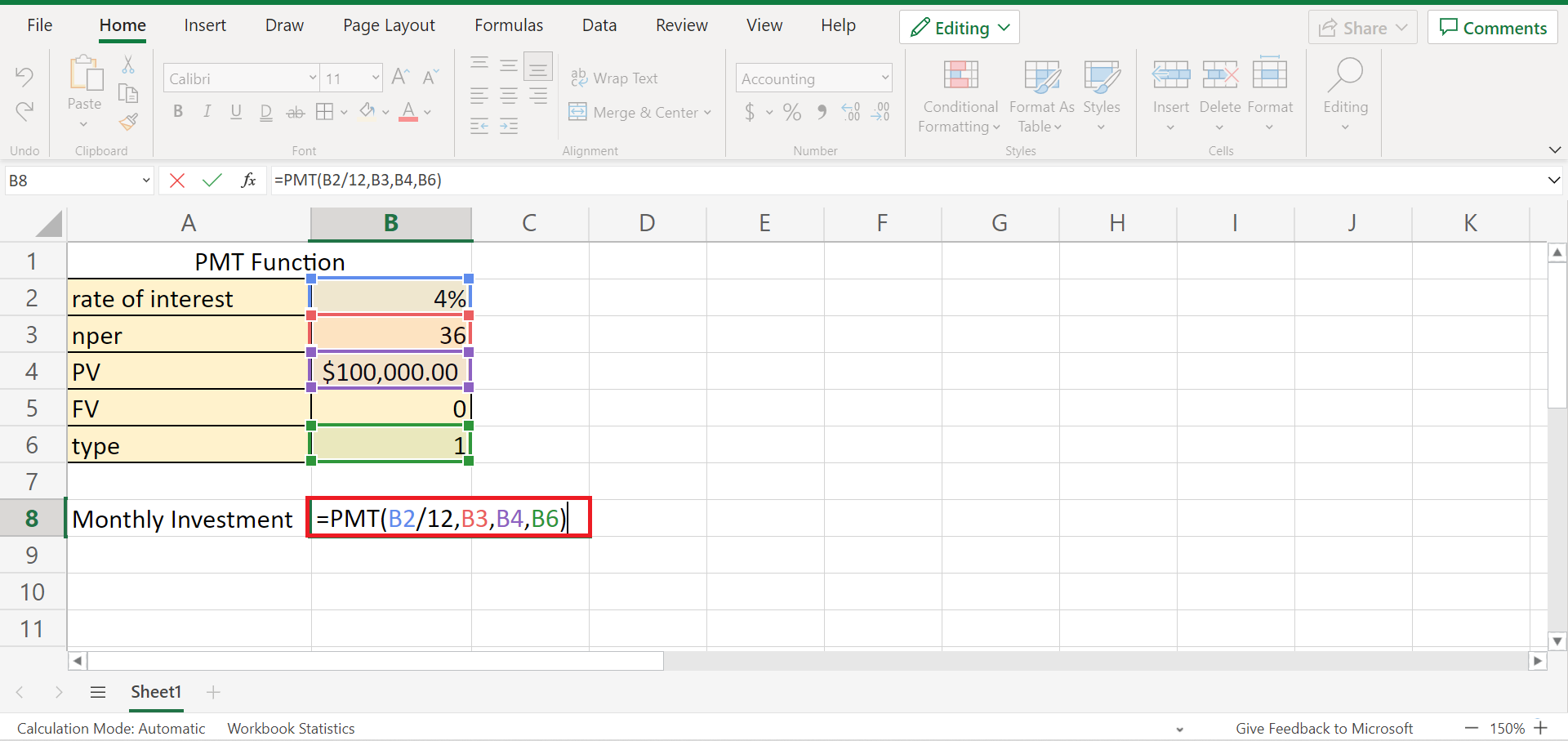# How to Use the PMT Function in Excel

This guide will explain how to use the `PMT` function in Excel.

The rules for using the `PMT` function in Excel are the following:

• When the given rate value is less than or equal to -1, the `PMT` function returns a #NUM! error.
• When the nper value is equal to 0, the `PMT` function returns a #NUM! error.
• When any of the arguments given is non-numeric, the `PMT` function returns a #VALUE! error.
• We need to convert the number of periods to months or annual interest rates to months or quarters.

Excel is an extremely useful tool containing several functions which can be used for several calculations and tasks. And one of these functions is the `PMT` function in Excel.

So the `PMT` function is used to calculate the payment for a loan based on constant payments and a constant interest rate. And `PMT` stands for payment. Furthermore, this function is mostly used for financial purposes to figure out payments for a loan.

Let’s take a sample scenario.

Suppose you took out a car loan. And you want to calculate how much you have to pay for your loan on a monthly basis. So this is where you can utilize the `PMT` function to simply and quickly get the monthly payment amount.

Great! Before we discuss a real example of using the `PMT` function in Excel, let’s first understand the syntax of the `PMT` function.

## The Anatomy of the PMT Function

The syntax or the way we write the `PMT` function is as follows:

`=PMT(rate, nper, pv, [fv], [type])`

Let’s take apart this formula and understand what each term means:

• = the equal sign is how we start any function in Excel.
• PMT() this is our `PMT` function. And this function is often used for financial aspects such as interest rates and calculating loan payments.
• rate is a required argument. And this refers to the interest rate per period for the loan.
• nper is also a required argument. And this refers to the total number of payments for the loan.
• pv is another required argument. And it refers to the present value, meaning the total amount the future payments are worth now.
• fv is an optional argument. And it refers to the future value you want to attain after the last payment is made. Additionally, this will be 0 if omitted.
• type is another optional argument. And it refers to a logical value or the payment made at the start of the period.

Awesome! Now let’s move on and discuss a real example of using the `PMT` function in Excel.

## A Real Example of Using the PMT Function in Excel

Let’s say we want to invest in the business. And assuming that we will receive an amount of \$100,000 after three years. In this case, our rate of interest is 4% per year, and the payment will be made at the start of each month.

So this is what the details of our `PMT` function would look like:Afterward, we can simply utilize the `PMT` function and input the necessary arguments based on the given values to obtain the amount for our monthly investments.

Lastly, we will obtain the final result, which is \$2,952.42. So this will be the monthly investment we need to pay at the start of each month.Great! Now let’s dive into the step-by-step process of how to use the `PMT` function in Excel.

## How to Use the PMT Function in Excel

In this section, we will explain the step-by-step process of how to use the `PMT` function in Excel. Furthermore, each step will contain a detailed explanation and pictures to guide you along the process.

1. Firstly, we must create a table of values for the necessary component to calculate the `PMT` function. Next, we will input the rate of interest, which is 4%. Then, our nper is 36 because we will only receive the money after 3 years. Next, we will input the present value, which is \$100,000.2. Secondly, we can now perform the `PMT` function in Excel. First, type an equal sign to start the function and type in the `PMT` function. Next, select the cell containing the rate of interest, nper, and PV. So our entire formula would be “=PMT(B2/12,B3,B4,B6)”. Lastly, press the Enter key to return the result.3. And tada! We have successfully used the `PMT` function to calculate the monthly investment amount.And that’s pretty much it! So we have discussed the rules of using the function, the syntax of the `PMT` function, and the steps on how to use the function in Excel. And now, you can apply this learning whenever you need to.

Are you interested in learning more about what Excel can do? You can now use the `PMT` function and the various other Microsoft Excel formulas available to create great worksheets that work for you. Make sure to subscribe to our newsletter to be the first to know about the latest guides and tutorials from us.Our goal this year is to create lots of rich, bite-sized tutorials for Google Sheets users like you. If you liked this one, you'll love what we are working on! Readers receive ✨ early access ✨ to new content.

##### You May Also Like## How to Calculate Probability in Excel

The PROB function in Excel is useful when you need to calculate for probability in Excel. This guide…## How to Group Columns in Excel

This guide will explain how to group columns in Excel using the built-in Group feature. Users can take…## How to Get Address of Named Range in Excel

The ADDRESS function is useful when you need to find the address of a named range in Excel.…## How to Filter Dates by Year in Excel

This guide will discuss how to filter dates by year in Excel using the filter tool.  Due to…